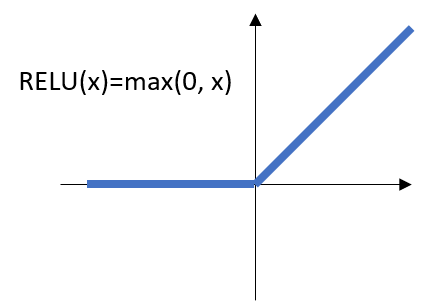# TensorFlow 筆記(4): 改善 simple logistic regression model

Source: Deep Learning on Medium# variable scope

`def build_model(X, num_input, num_output): n0 = num_input # MNIST data input (img shape: 28*28) n1 = 256 # number of neurons in 1st hidden layer n2 = 256 # number of neurons in 2nd hidden layer n3 = num_output # output layer W1 = tf.Variable(tf.random_normal([n0, n1])) B1 = tf.Variable(tf.random_normal([n1])) W2 = tf.Variable(tf.random_normal([n1, n2])) B2 = tf.Variable(tf.random_normal([n2])) W3 = tf.Variable(tf.random_normal([n2, n3])) B3 = tf.Variable(tf.random_normal([n3])) Y1 = tf.add(tf.matmul(X, W1), B1) Y2 = tf.add(tf.matmul(Y1, W2), B2) yhat = tf.add(tf.matmul(Y2, W3), B3)`

`get_variable` 的作用是當 variable 存在時就取出 variable, 若 variable 不存在則創建 variable